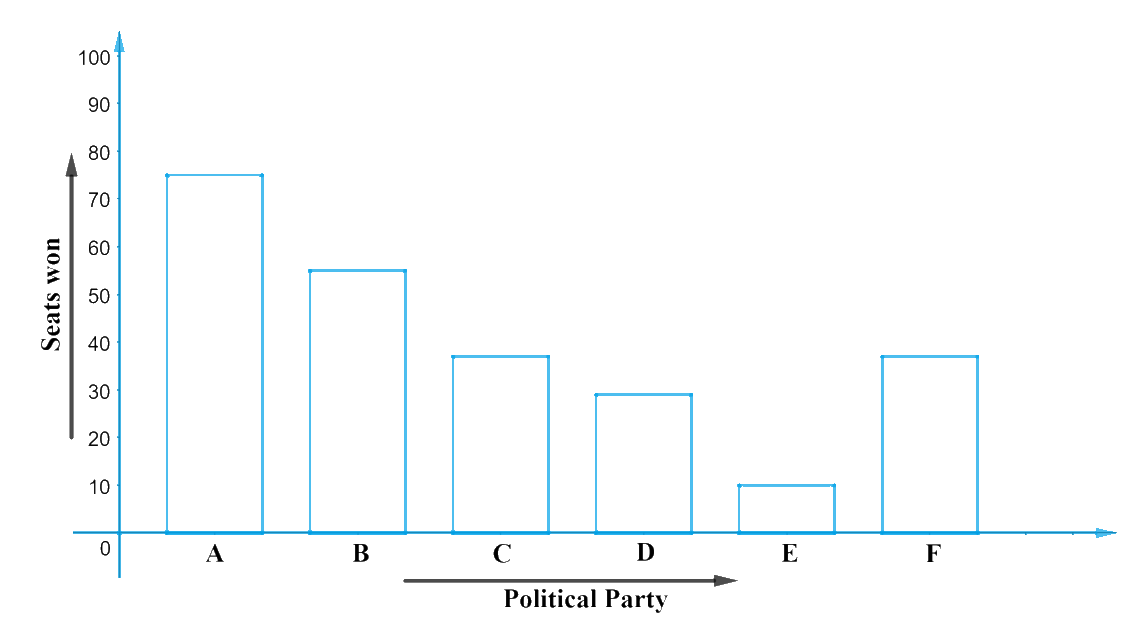# Ex.14.3 Q3 Statistics Solution - NCERT Maths Class 9

Go back to  'Ex.14.3'

## Question

Given below are the seats won by different political parties in the polling outcome of a state assembly elections:

 Political Party $$A$$ $$B$$ $$C$$ $$D$$ $$E$$ $$F$$ Seats Won $$75$$ $$55$$ $$37$$ $$29$$ $$10$$ $$37$$
1. Draw a bar graph to represent the polling results.
2. Which political party won the maximum number of seats?

Video Solution
Statistics
Ex exercise-14-3 | Question 3

## Text Solution

What is known?

The seats won by different political parties in the polling outcome of a state assembly elections.

What is unknown?

1. Bar graph to represent the polling results.
2. The political party which won the maximum number of seats.

Reasoning:

The given data can be represented through a bar graph by:

1. Taking ‘Political Party’ on $$x$$-axis and seats won on $$y$$-axis with a scale of $$1\,\rm unit = 10$$ seats on $$y$$-axis.
2. Maintaining equal width for all the bars in $$x$$-axis with equal gaps in between them.From the graph, it can be observed that the political party ‘$$A$$’ won the maximum number of results.

Learn from the best math teachers and top your exams

• Live one on one classroom and doubt clearing
• Practice worksheets in and after class for conceptual clarity
• Personalized curriculum to keep up with school